## [Work in Progress DRAFT VERSION Based on notes from 09 and 11]

The use of Java has become a browser and machine dependent issue.
In particular, the Java used in David Joyce's version of Euclid does not work uniformly in Firefox on HSU computers, but does seem to work on Chrome. Other Java works in Firefox, but not in Chrome. I will try to indicate these dependencies when possible.
Readers who might have difficulty running the Java applets are advised to use a book marklet that converts JavaSketchpad sketches on this page (or anywhere else on the internet) to work completely independently of Java.
Please give feedback, whether bug reports or other, at the following email address: wsp@kcptech.com
 Week Monday Wednesday Friday 1 1-20  Introduction 1-22 Continue discussion of what is "geometry"?  Start on Euclid- Definitions, Postulates, and Prop 1. 2 1-25 Euclid- Definitions, Postulates, and Prop 1. cont'd 1-27 Pythagorean plus. 1-29 Euclid Postulates/ Pythagoras 3 2-1 Euclid early Props/ Pythagoras/ 2-3 Euclid early Props/ Pythagoras/ 2-5 Equidecomposable polygons 4 2-8 More on Details for triangulation of planar polygonal regions  and adding parallelograms. 2-10 Begin Constructions and the real number line  M&I's Euclidean Geometry 2-12 Constructions from M&I. 5 2-15   Begin Constructions and the real number line. 2-17 Similar Triangles.Intro to Proportions. Construction of rational numbers.  Constructions and  The real number line.- Continuity 2-19 Coordinate based proofs. Inversion and Orthogonal Circles . Isometries:Classification of Isometries 6 2-22 Inversion and Orthogonal Circles 2-24 Continuity applied.. Isometries:Groups and Classification of Isometries. 2-26 Convexity of intersections. Start Proof of classification result for plane isometries 7 2-29 Finish Classification of Line Isometries and coordinates 3-2 Finish Classification of Plane Isometries and coordinates 3-4 Isometries and symmetries Begin Affine Geometry 8 3-7 Proportion and Similarity 3-9 Euclidean (Eudoxus) Proportion. . 3-11 Euclidean (Eudoxus) Proportion.and similarity, 9 3-14 No class Spring Break! 3-16 No class Spring Break! 3-18 No class Spring Break! 10 3-21 Euclidean Proportion and ratios of lengths with numbers. Central Similarity as a transformation. 3-23 The Affine Line and Affine Geometry (planar coordinates). Affine geometry- Homogeneous coordinates for aa affine line 3-25 The Affine Line and Affine Geometry (planar coordinates). Affine geometry- Homogeneous coordinates and Visualizing the affine plane. 11 3-28 Watch Non-Euclidean Universe (Open Univ. Video)- Lib. 3-30 The 7 point Geomoetry. Axioms and Stuctures 4-1 Watch Conics (Open Univ. Video)- Lib. 12 4-4  Affine geometry- Homogeneous coordinates and Visualizing the affine plane. 4-6 More on Affine Projective Geometry - Algebra! 4-8 Desargues! 13 4-11 Review and start of Synthetic Projective Geometry 4-13 More Review and start of Synthetic Projective Geometry 4-15 RP(2) 14 4-18 Proofs - planes in Projective Geometry 4-20 A start on transformations with homogeneous coord.s. The Planar Duality Principle 4-22 Complete quadrangles. 3 dimensions, Sections and perspectivities. 15 4-25 Projectivities and harmonics 4- 27 4-29 16 5-2 5-4 5-6

• 1-20/22 Introductory Classes Summary (+).
What are different aspects of geometry? How is the study of geometry organized? Analytic(numbers) , Synthetic(axiomatic),  Transformations (functions) are three ways to organize information and the study of geometry. Also Projective and Differential geometry were mentioned as alternative focuses for studying geometry.
• Different types of geometry:
• Euclidean: Lengths are important
• Similarity: Shape is important
• Affine: Parallel lines are important.
• Differential: Curvature is important.
• Topological: General shape- especially holes and connectedness- is important.
• What is synthetic geometry? A geometry that focuses on connecting statements (theorems, constructions) to a foundation of "axioms" by using proofs.
• What is analytic geometry? A geometry that focuses on connecting statements (theorems, constructions) to a foundation of number based algebra.
• What is "structural geometry"? A geometry that focuses on connecting statements (theorems, constructions) to a foundation of structures (relations and operations) on sets by using proofs.
• transformations: tools that allow for changing figures:[the basis for studying different geometries in the Klein Erlangen Program. See Felix Klein - YouTube Felix Klein (25 April 1849 – 22 June 1925)
• Euclidean: translations, rotations, and reflections.
• Similarity: magnifications, dilations
• Affine: Preserve parallel lines
• Projective:  "linear projections"...line preserving
• Differential:   "smooth".
• Topology: continuous
1-25     Still to be covered is the course project on the assignments webpage.
• geometry as an empirical science
• geometry as a formal system of information
• geometry focused on special objects like triangles, or special qualities like convexity.
• Geometry on the web and using GeoGebra

• Geometry has traditionally been interested in both results- like the Pythagorean Theorem- and foundations -  using  axioms to justify the result in some rigorous organization. We will be concerned with both results and foundations.
• We will attempt to build an understanding of both the synthetic and analytic approaches to geometry by using the tools of a structural approach.

•  In the distinction between synthetic and analytic geometry the key connecting concept is the use of measurements.
• Initially we will try to avoid when possible the use of measurement based concepts in the structures we use .

• In one alternative proof for this theorem illustrated in the GeoGebra sketch below, follow the movie to consider 4 congruent right triangles and the square on the side of the hypotenuse arranged inside of a square with side "b+c" and then moving through the movie the same 4 triangles and 2 squares arranged inside of a square with side "b+c"  . Can you explain how this sketch justifies the theorem?
• 1-27 Review of previous "proof of the PT.

•   Consider the on-line version of Euclid's Geometry- especially Book 1:
• The list (on-line) of initial definitions and postulates. ( We will continue a discussion of these in more detail later in the course.) The end results of Book I are The Pythagorean Theorem and its converse.
• Consider Proposition 1 and that statement appear justified by the definitions and postulates! A more detailed discussion of this proposition is found below.

• Discuss: What would we need in geometry to make a proof of the PT based on this activity?
• What kind of structures and assumptions were needed in the proof with the 4 triangles and squares?

Here are some considerations related to those assumptions:
• What types of objects are being considered?
• points
• lines
• line segments
• angles
• triangles
• squares
• What types of relations are being considered?
• points on lines
• Lines intersecting at points
• Congruent line segments
• congruent angles
• congruent figures (triangles, squares)
• Decomposing and recomposing a figure.
• How could we justify identifying "equal" objects (congruent figures)?
• How do the objects fit together?
• How do movements effect the shapes of objects.
Consider the Pythagorean Theorem Activity Sheet.

• Back to Euclid for some further examination of the postulates:
• Looking at the definitions and postulates, a key feature of any geometric structure appears early- What defines a point and a line?
• An alternative- minimal geometry:  points: A,B.  lines: {A,B}. That's all!
• Postulate 1. To draw a straight line from any point to any point.
• Current view:  Two distinct points determine a unique straight line.
• Issue: What is a "straight" line?
• Alternative geometry- spherical. Points: points on a unit sphere in coordinate space: { (x,y,z): x2 +  y2 +  z2=1}
Straight lines:  Great Circles on the sphere. Determined by a plane in space through (0,0,0).
Fact: Two points on the sphere that are not antipodal- (Opposite) determine a unique straight line. [Use the plane determined by the two points and (0,0,0).] For this to make this spherical geometry satisfy Post 1- redefine what "distinct" means: If the points on the unit  sphere are antipodal, then they are considered "equal" and thus not distinct.
1-29:

• Postulate 2. To produce a finite straight line continuously in a straight line.
• Current view: A straight line has no "endpoints".
• Issue: What is the nature of a straight line not being "finite"?
Alternative Geometry.  Points: all numbers in an open interval {x: 0<x<1}. Straight line: The interval (0,1) and all subintervals.
A "finite" straight line: Any closed sub-interval of (0,1).
Fact: Any closed sub-interval of (0,1) is properly contained in another closed subinterval of (0,1).
Proof: Suppose 0<a,b<1. Then [a,b] is contained in the interval [a/2, (b+1)/2] which is contained in (0,1).
• Postulate 5. That, if a straight line falling on two straight lines makes the interior angles on the same side less than two right angles, the two straight lines, if produced indefinitely, meet on that side on which are the angles less than the two right angles.
• Current view: Under the given circumstances, the two straight lines are not parallel.
Issue: How can you find the actual point where the lines meet?
Here is the most distinctive of the postulates that appear in Euclid.
The definition of parallel: Parallel straight lines are straight lines which, being in the same plane and being produced indefinitely in both directions, do not meet one another in either direction.
• Looking at "Common Notions" we see the geometry has language for equality and addition- a relation and an operation for the objects.

• 2-1
• Look at how the definitions and postulates are used in Euclid:
• These propositions demonstrate that Euclid did not treat moving a line segment as an essential property worthy of being at the foundations as an axiom. However, this is a fundamental tool  for all of geometry.
• Note that in the proof of proposition 1 the point of intersection of circles is presumed to exist without reference to any of the postulates. This presumptions was left implicit for hundreds of years, but were cleared up in the late 19th century (see the work of David Hilbert) when careful attention was given again to the axioms as a whole system.

•  Notes on Existence: Existence is an often overlooked quality in a mathematical statement:
For example it is common to state that sqrt{2} is an irrational number.
The proof starts by assuming sqrt{2} is a rational number,  say sqrt{2} = p/q where p and q are natural numbers, so that ... 2 q^2 =  p^2. From this the usual proof of the statement deduces a contradiction. However, the statement assumes that there is a number the square of which is equal to 2.
One can justify the existence of the square root of 2 in many ways. One way presumes that any line segment has a length measured by a real number. Then by the Pythagorean theorem, the diagonal of a unit square will have length sqrt{2}.

• Alternative "rational coordinate" plane geometry.  [A geometry in which circles do not always intersect.]
• Points: ordered pairs of rational numbers.

An example of a point not in the rational coordinate plane is the point (sqrt{2},0). This point can be constructed in the ordinary plane with straight edge and compass using the circle with center (0,0) and radius determined by the points (0,0) and (1,1). This circle will meet the X-coordinate axis at the point (sqrt{2},0)

The circle with center (0,0) and radius 1 and the circle with center (1,0) and radius 1 meet in the ordinary plane at the points with coordinates (1/2, sqrt{3}/2) and (1/2, -sqrt{3}/2) . Since sqrt{3}/2  is not a rational number, this ordered pair does not correspond to a point in the rational coordinate plane, so the two circles do not have a point of intersection in the rational coordinate plane.

• Note: Proposition 1 has a statement that was not justified by the definitions and postulates!
• Proposition 2 demonstrates a concern of Euclid about establishing a "motion" or transformation for this geometry based on fundamental postulates.
• An example of the "web" of information formed in this "axiomatic" synthetic geometry.
• Not just demonstrations- but the first two propositions are dedicated to constructions! A focus on existence as well as conditions that guarantee other conditions.

2-3 Some Comments on Problem Set #1:Intersections for families of convex figures:
Using the notation of M  & I:
cap \{ [P_0P_r : r>0\} = \{P_0\} ; cap \{ [P_{-1-r}P_{1+r} : r>0\} = \{P_{-1}P_1\}
To show that \D = {P in plane where d(P,P^*) le 1} is convex where P^* is a point in the plane,
recognize that D = cap { half planes determined by tangent lines to the boundary of D that contain P^*}. These half planes are all convex.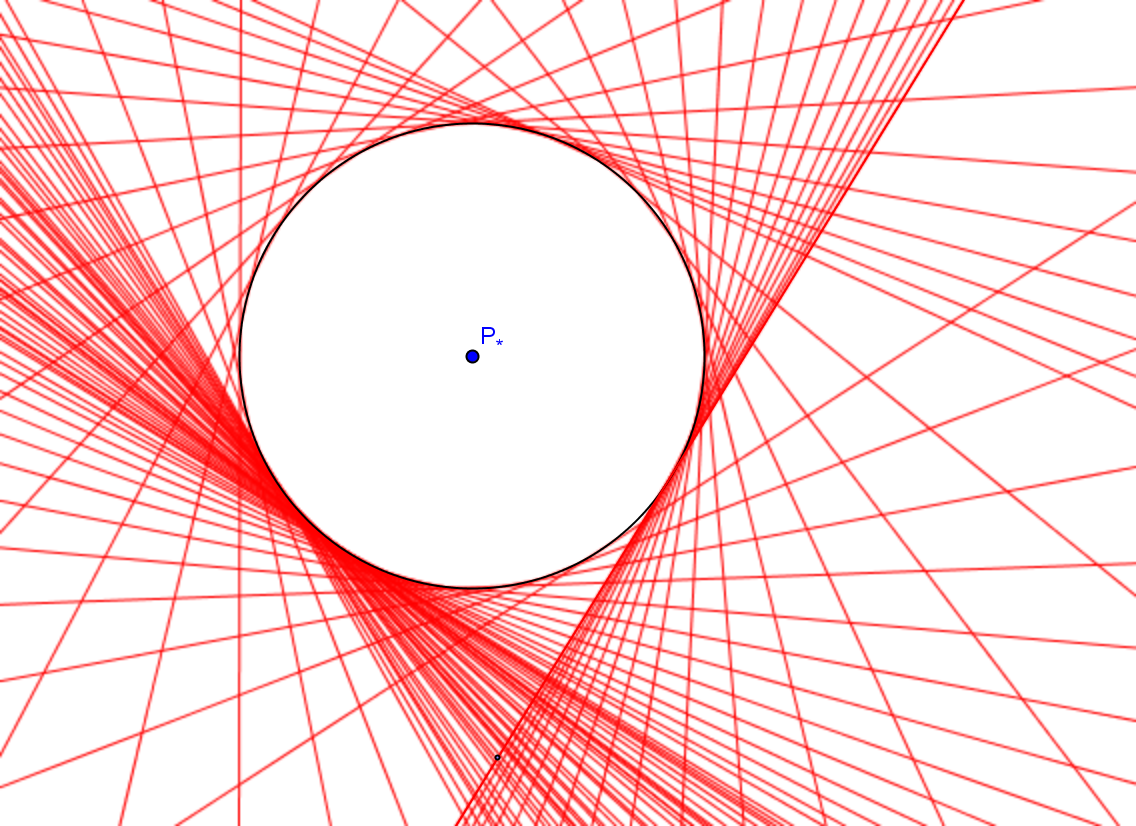• Note that M&I build an informal presentation of a geometry structure based on using the real numbers.
• To explore some of these issues, let's looked at  the Pythagorean Theorem and its proofs.
• In right-angled triangles the square on the side opposite the right angle equals the sum of the squares on the sides containing the right angle.

Note that Euclid's treatment in its statement or its "proof" never refers the traditional equation, a2+b2=c2.

• Measurement and the Pythagorean Theorem (PT)
•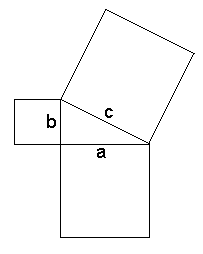a2 + b2 = c2

Another proof using "shearing" illustrated in the GeoGebra visual proof  by choosing "Shear Pythogoras"and the Java sketch below taken from a Geometers' Sketchpad example that can be connected to Euclid's proof.

(Based on Euclid's Proof)  D. Bennett 10.9.9
1. Shear the squares on the legs by dragging point P, then point Q, to the line. Shearing does not affect a polygon's area.
2. Shear the square on the hypotenuse by dragging point R to fill the right angle.
3. The resulting shapes are congruent.
4. Therefore, the sum of the squares on the sides equals the square on the hypotenuse.

[Side Trip?] Moving line segments: Can we move a line segment without changing its length.

2-5
We can look further at the foundations of the proofs of the Pythagorean Theorem in two ways:
1. Dissections: How are figures cut and pasted together? Can be the proof be achieved using dissections?
Solution!

2. Transformations: How are figures transformed? What transformations will leave the measurements of  "area", "lengths", and "angle measures" of figures invariant (unchanged)?

A look at the possibilities of dissections .

Puzzles and Polygons
• Tangrams. More
• Tangrams – Use all seven Chinese puzzle pieces to make shapes and solve problems.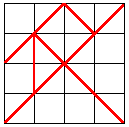• Cutting and reassembling polygons. More ....
• Dissections (like Tangrams) and equidecomposable polygons.
• Use tangram pieces to make a square.
• Note that in putting the pieces together to form any other shape, the area of that shape would be the same as the area of the square unless there is some overlap of the pieces in the shape.
• Question: Is this necessary condition of equal areas sufficient to say that two polygonal regions could be decomposed (cut and pasted) into smaller regions that would be congruent?

• Comment: In a sense a positive (yes) response to this question means that one could create a set of smaller shapes with which one could make either of the two regions using precisely these smaller shapes. The answer to this question is yes (in fact this is a late 19th - early 20th century result), which is the basis for the remainder of this section
[The analogous problem in three dimensional geometry: volume equality of polyhedra is a necessary but not sufficient condition for a similar result. This was the third of the famous 23 "Hilbert problems" for the 20th century. This was first demonstrated by Dehn almost as soon as the problem was announced. Dehn used another invariant of polyhedra related to the lengths of the edges and the dihedral angles between the faces of the polyhedra.[ "Ueber den Rauminhalt," Math. Annalen, 55 (1902), 465-478].

See also A New Approach to Hilbert's Third Problem - University of ...by D Benko.
First, consider some of the background results which were known to Euclid: (1) parallelograms results and (2) triangle results. The justifications for these results can be  reviewed briefly.

(1) a. Parallelograms between a pair of parallel lines and on the same line segment are equal (in the sense of being able to decompose one to reconstruct the other).  Proposition 35.
b. Parallelograms between a pair of parallel lines and on  congruent segments are equal (in the sense of being able to decompose one to reconstruct the other). Proposition 36.

(2) a. The line segment connecting the midpoints of  two sides of a triangle is parallel to the third side and is congruent to one half of the third side.
[The justification of this result is left as an exercise in traditional Euclidean Geometry.]
b. By rotating the small triangle created by connecting the midpoints of two sides of a triangle 180 degrees about one of the midpoints, we obtain a parallelogram. (This shows that the triangle's area is  the area of this parallelogram which can be computed by using the length of the base of the triangle and 1/2 of its altitude- which is the altitude of the parallelogram.)
Compare this with Euclid  Prop. 42 and Prop. 44.

2-8

(ii) The triangulation of any polygonal region
in the plane is a key element in a proof of the equidecomposable polygon theorem.
The proof of this proposition examines a more careful characterization of the polygonal regions being considered. The key idea of the proof goes by induction on the number n = the number vertices = the number of sides in the polygon.  Proof of the triangulation proposition.
Another component of the proof of the equidecomposable polygon theorem is the ability to "add two parallelograms to form a single parallelogram which is scissors congruent to the two separate parallelograms". Here's how:

 Intersect two pairs of parallel lines, l and l' with m and m'- one from each of the given parallelograms. Draw a diagonal HI in the resulting parallelogram. Cut and translate one parallelogram so that it is scissors congruent to a parallelogram HIJK within the same parallel lines l and l' with one side being the diagonal. Cut and translate the other parallelogram so that it is scissors congruent to a parallelogram HINO within the same parallel lines m and m' with one side being the diagonal and on the other side of the diagonal HI from the transformed first parallelogram. Now draw the parallel NO to the diagonal in the second transformed parallelogram HINO so that it intersects the parallels  l and l' from the first parallelogram at the points P and Q. This makes one larger parallelogram JKPQ which is scissors congruent to the original two parallelograms. Compare this with  Euclid Proposition  45. This is a Java Applet created using GeoGebra from www.geogebra.org - it looks like you don't have Java installed, please go to www.java.com

Follow this link for a proof of the equidecomposable polygon theorem. or here is a slightly different approach.

The film Equidecomposable Polygons also proves the result:
If two polygonal regions in the plane have the same area, then there is a decomposition of each into polygons so that these smaller polygons can be moved individually between the two polygons by translations or half turns (rotations by 180 degrees).

2-10
Footnote on Equidecomposable Polygon Theorem:
The relation of being "scissors congruent" (sc) is an equivalence relation:
1.  A   sc  A
2. If A sc B then B sc A.
3. If A sc B and B sc C then A sc C.
The theorem can be interpreted by saying for any A and B, polygonal regions in the plane, A sc B if and only if Area(A) = Area(B).

General discussion of invariants:
Geometry (and Math in general) studies objects and how they are transformed.
Some features of the objects are preserved by the transformations. These features are often described as invariants of the transformation.
In studying  equidecomposable polygons, the objects are polygonal regions in the plane, and the transformations are scissors congruences.
The area of the initial polygon is the same as the area of the transformed polygon, so area is an invariant of scissor congruence.
The general question: Given some objects and transformations, what are the invariants of these transformations?  Is there a collection of invariants for the transformations so that if two objects have the same invariants then they are equivalent in some sense based on the transformations being studied.
The main impact of the Equidecomposable Polygon Theorem: For planar polygons and scissors congruence, the area of the polygon is an invariant that is sufficient to determine when two polygons are or are not scissors congruent
.
Another geometric relation connected to transformations is congruence for triangles.
We will study further the transformations that are key to the congruence relation: translation. rotation, and reflection.
There are many invariants for these transformation: they include the length of segments and the measurement of angles as well as area of the triangles.
It should be clear that the area of two triangles is not enough to determine whether they are congruent.
There are some key invariants of a triangle which can determine congruence:
• The three corresponding sides of two triangles are congruent: SSS
• Two sides and the included angle of two triangles are congruent: SAS
• Two angles and the included side of two triangles are congruent: ASA.

Read  the definitions in M&I section 1.1

REVIEW of basic plane geometry concepts and definitions based on M&I.
M&I build their foundations for Euclidean geometry on a one to one correspondence between points on a line and real numbers and the ability to match angles with numbers between 0 and 180.

Review materials defining  rays, segments, angles, triangles, and planes  in M&I.
These definitions present a model for geometry based on the real numbers. Points, rays, line segments, etc., are identified with real numbers and intervals of real numbers. Notice that angles in the plane are not oriented and  are always considered to have a measure between 0 and 180.

2-12
Review eight of the basic Euclidean constructions described in M&I section 1.2.
Note that several of these constructions rely on some foundations that assert the existence of points of intersection of circles. Thus these constructions will not be guaranteed to be effective in a geometric structure where such points do not exist- such as the geometry of the rational number plane.
 Angle Bisection Euclid Prop 9 Line Segment Bisection Euclid Prop 10 Construct Perpendicular to line at point on the line Euclid Prop 11 Construct Perpendicular to line at point not on the line Euclid Prop 12 Move an angle Euclid Prop 23 Construct Parallel to given line through a point Euclid Prop 31

Some comments about Constructions: It is important to notice that constructions also require a justification (proof) that the construction has in fact been achieved.

In proving the constructions we use some basic euclidean results, such as the congruence of all corresponding sides in two triangles is sufficient to imply the triangles are congruent (SSS). [Other basic Euclidean results are SAS and ASA congruence conditions, as well as the result that corresponding parts of congruent triangles are congruent (CPCTC).]

For these particular constructions to be justified by the same arguments given by Euclid in a geometric structure, the structure will need both an equivalence relation called congruence for line segments, angles, triangles and propositions that connect congruence of triangles to sufficient conditions  like  SSS, SAS, and ASA.

The constructions play two important but different roles in (euclidean) geometry:

(i) Construction allow us to "move", deconstruct and reconstruct figures, while maintaining the magnitudes of the pieces and angles . Thus constructions provide the tools for transformations such as rotations and translations.
(ii) Constructions allow us to develop comparative measurements based on a "unit" segment and the "straight" angle.

It should also be noted that the three transformations (translation, rotation, and reflection) commonly used in geometry are connected to constructions as well. For example, to translate a figure by a vector it would be useful to know how to construct parallelograms.
We reviewed the connection between the Euclidean constructions and the three transformations (translation, rotation, and reflection) and the fact that if T is either a translation, determined by a vector ( an oriented line segment), a rotation determined by a center and an oriented angle, of a reflection, determined by a line, the image if a triangle T( Delta ABC) = Delta A'B'C'  is a  triangle that is congruent to  Delta ABC.
Other properties of these transformations, that preserve measure of line segments and congruence of angles, as well as similarities will be discussed further.

Note on midpoints: With the construction of midpoints in Euclidean Geometry, we can show that a Euclidean line segment has an infinite (not finite) number of distinct points. Furthermore, if we think of approximating real number distance with points on a segment after establishing a unit length, then we can construct the position of a euclidean point as close as we want to the position where a real number might correspond to a point in that position.

• The construction of points that correspond to numbers on a line.

• First construct P_k where k is an integer using circles.
We can construct points with fractions using powers of 2 for the denominator by bisection.
(However, with only bisection we could not construct a point for 1/3 although we could get very close to that point using a binary representation of that common fraction.) Using  midpoints of segments we can construct points that correspond to n/(2^k) for any n and k. Unfortunately real numbers like sqrt{2} and pi  cannot be expressed in this way. To obtain a correspondence of points with all real numbers using bisection we need a geometric property that will give a point to correspond to any convergent sequence of numbers of the form n/(2^k).

The continuity axiom for a euclidean line:[Cantor's Axiom]
• Any non empty family of nested segments will have at least one point in the intersection of the family.
This axiom allows us to make a 1 to 1 correspondence between any real number and a point on a euclidean line once points have been determined to correspond to 0 and 1.

I.e., given P0 and P1   for any real number x where a<x<b there is a point Px where Px is between Pa  and Pb if and only if  the point P_{n/(2^k)} corresponds to the number n/(2^k)  for any integer n and natural number k .

[Review the construction of a line though a given point parallel to a given line. See Euclid  I.31.]
Brief Introduction to Similar Triangles: [More details later in the course.]
Basic Result: Given Delta ABC and Delta A'B'C' with <Acong<A' and  <B cong <B' then <Ccong<C' and {m(AB)}/{m(A'B')} = {m(BC)}/{m(B'C')} = {m(AC)}/{m(A'C')} and we say that Delta ABC is similar to  Delta A'B'C' and write Delta ABC ~ Delta A'B'C'.
This fact is used repeatedly in M&I to justify the construction of P_{k/n}, a rational point on the number line for the rational number k/n from an integer lattice on the euclidean plane.
We can construct P_{k/n} when k/n is a rational number using the theory of similar triangles.

For example: We can bisect or trisect a line segment, giving us the ability to find points representing rational numbers with denominators involving powers or 2 and 3, such as 5/6, 7/18, etc.
The figure below gives two ways to achieve these constructions. One can see how to generalize these to allow one to construct points to represent any rational number on the line so that the arithmetic of numbers is consistent with the arithmetic of geometry. [Adding segments and adding numbers, etc.]

Consider M&I's constructions of the same correspondence of integer and rational points. These also rely on the ability to construct parallel lines.

Using the rational number points and the continuity axiom for a euclidean line we can construct a unique point P_x on the euclidean line for every real number x by using the (infinite) decimal representation of the number. With this correspondence the order relation of the real numbers corresponds to the between-ness relation of points on a line segment and there is a geometric construction for the arithmetic operations with real numbers that matches all the arithmetic properties of the real numbers.
For example we can "add" P_x to P_y to obtain a new point P_s and s = x+y. This can be done with circles or with parallel lines.
We will look at multiplication and division further in the next few sessions. Historically this "isomorphism" between the real number arithmetic and geometry constructions was identified by Descartes in his work The Geometry.(1637)

2-19
Examples of using coordinates  (and Vectors) for proofs in euclidean geometry:

Proposition: An angle inscribed in a semicircle is a right angle.
Restated: If AC is the diameter of a circle and B is a distinct point on the circle, then <ABC is a right angle.

Coordinate Proof: Using coordinates, assume the center of the circle has coordinates (0,0) and that the point A has coordinates (1,0) and C has coordinates (-1,0) while B has coordinates
(x,y) where x^2 + y^2 = 1.
To show <ABC is a right triangle by application of the converse to the Pythagorean theorem it suffices to show that [(x - 1)^2 + y^2 ]+ [(x + 1)^2 + y^2] = 2^2 = 4.
This can be verified by expanding the squared terms involving x giving :   [x ^2 + 1  + y^2 ]+ [x ^2  + 1 + y^2] = 2+2 = 4. EOP.

Vector Proof: Let v be the vector from A to C and w be the vector from B to C. It suffices to show the dot product of these vectors is 0.
Now v cdot w =  ( (x - 1)(x+1) + y^2 ] = x^2 - 1 + y^2  = 0. EOP.
Introducing Orthogonal Circles and The inverse of a point with respect to a circle. Convexity of a geometric figure.

Tangents to circles.
In considering constructions of tangents to circles we use the characterization of a tangent line as making a right angle with a radius drawn at the point it has in common with the circle. ( Book III Prop. 16.) In our construction, not Euclid's (Book III Prop. 17), we also use the result that any angle inscribed in a semi-circle is a right angle. ( Book III Prop. 31.)

Note on Three Historical Problems of Constructions with Straight Edge and Compass (Euclidean Constructions)
(1) Trisection of an angle: Since it possible to bisect and trisect any line segment and bisect any angle, the issue is, is it possible to trisect any angle?
Note: If you use folding and Origami construction it is possible to trisect an angle. [How to Trisect an Angle with Origami - Numberphile ...]

(2) Duplication of a cube [Wikipedia]: Since it is possible to construct a square with twice the area of a given square, the issue is, is it possible to construct a cube with twice the volume of a given cube?

(3) Squaring a circle: Since it is possible to construct a square the same area as any given polygonal region, the issue is, is it possible to construct a square with the same area as a given circle.
[Squaring the Circle]

Doing arithmetic with constructions in geometry. Note that the construction above allows one to construct a point Px' from a point Px as long as x is not 0 so that x' x = 1.[ Use the circle of radius 1 with center at P0 to construct the inverse point for Px.]

2-22

The relation of the inversion transformation with respect to a circle and orthogonal circles.
Proposition: If C2 is orthogonal to  C1  (with center O) and A is a point on C2 then the ray OA will intersect C2 at the point A' where A and A' are inverses with respect to the circle C1. Click here for the proof.

We can use this proposition in the following
Constructions: 1. Construct a circle C2 through a given point B on a circle C1 and a point A inside the circle so that C2 is orthogonal to C1.

Solution: First construct the inverse A'  of A with respect to C1 and then the tangent to C1 at B and the perpendicular bisector of AA' will meet at the center of the desired circle.

2. Construct a circle C2  through two points A and B inside a circle C1 so that C2 is orthogonal to C1.
Solution: This solution is demonstrated in the sketch below.

2-24
Further notes on the continuity axiom.

The continuity axiom leads to the important result due to G. Cantor that  any list (possibly infinite) of points in a given segment of a euclidean line will not have every point in that segment on the list. [Note  that we can make a list of points corresponding to the rational numbers once a unit length had been established.  1/1,1/2,2/1,1/3,2/2,3/1,1/4,2/3,3/2,4/1, ....]

The continuity axiom can also be used to prove: If a line, l, (or circle, O'A') has at least one point inside a given circle OA and one point outside the same given circle then there is of a point on the line (circle) that is also on the given circle.

Proof outline for the line-circle: Use bisection between the points on the line l outside and inside the circle OA to determine a sequence on nested segments with decreasing length approaching 0. The point common to all these segments can be shown to lie on the circle OA.

Proof outline for the circle-circle: Draw the chord between the inside and outside points on the circle O'A'. Use bisection on this chord to determine rays that by the previous result will meet the circle O'A'. The bisections can continue to determine a sequence of nested segments with decreasing length approaching 0 and with endpoints determining one outside point and one inside on O'A' . The point common to all the endpoints on the chord  will determine a point on O'A' that can be shown to also lie on OA.

Note: The circle-circle result fills in the hole in the proof of Proposition 1 in Book I of Euclid.

Isometries:

Definition: An isometry on a line l /plane π /space S is a function (transformation), T, with the property that for any points P and Q,  d(T(P),T(Q))=d(P,Q) or m(PQ)=m(P'Q').

Comment: Other transformation properties can be the focus of attention- replacing isometries as the key transformation in a geometrical structure. The connection between figures and the selected  transformations is made by the fact that the transformations have a "group" structure under the operation of composition. When the transformations form a "group" there is a resulting equivalence relation structure on the figures of the geometry. This is illustrated by the relation between isometries and "congruence" in euclidean geometric structures in which distance and/or measure has a role.

Facts: (i) If  T and S are isometries then ST is also an isometry where ST(P) = S(T(P))= S(P')  [T(P)=P'].

(ii) If T is an isometry, then the transformation S defined by S(P') = P when T(P) = P' is also an isometry.

Proof : (i) Consider d(ST(P),ST(Q)) = d( S(T(P)),S(T(Q)) ) = d(S(P'),S(Q')) = d(P',Q') = d(P,Q) .

(ii) Left as an exercise for the reader.

Comment: If G ={ T: T is an isometry of a structure}, then since Id(P)=P is an isometry, Facts (i) and (ii) together the fact that composition (product) of transformations is an associative operation make G together with the operation of composition a group structure.

2-26
Convexity: Another brief side trip into the world of convex figures.

Recall the Definition: A figure F is convex if whenever A and B are points in F, the line segment AB is a subset of F.

The half plane example: Consider the half plane determined by a line l and a point P not on the line. This can be defined as the set of points Q in the plane where the line segment PQ does not meet the line l.  Discuss informally why the half plane is convex.

Review the problems on convex figures in Problem Set 1.

Other convex examples: Apply the intersection property [The intersection of convex sets is convex.] to show that the interior of a triangle is convex.

Show that the region in the plane where (x,y)  has y>x^2 is convex using the tangent lines to the parabola y=x^2 and the focus of the parabola to determine a family of half planes whose intersection would be the described region.

Some General Features of Isometries:

What information determines an isometry?

Proposition: For a planar isometry, T, where T(P) = P', when we know T(A), T(B), and T(C) for A,B, and C three noncolinear point , then T(P) is completely determined by the positions of A', B', and C'.

Proof: In fact we saw that T(B)=B' must be on the circle with center A' and radius= m(AB), and  T(C)= C' must be on the intersection of the circles one with center at A' and radius = m(AC) and the other with center at B' and radius = m(BC). Once these points are determined, then for any point P, P' must be on the intersection of 3 circles, centered at A', B', and C' with radii = to m(AP), m(BP), and m(CP) respectively. These three circles do in fact share a  single common point because the associated circles with centers at A,B, and C all intersect at P.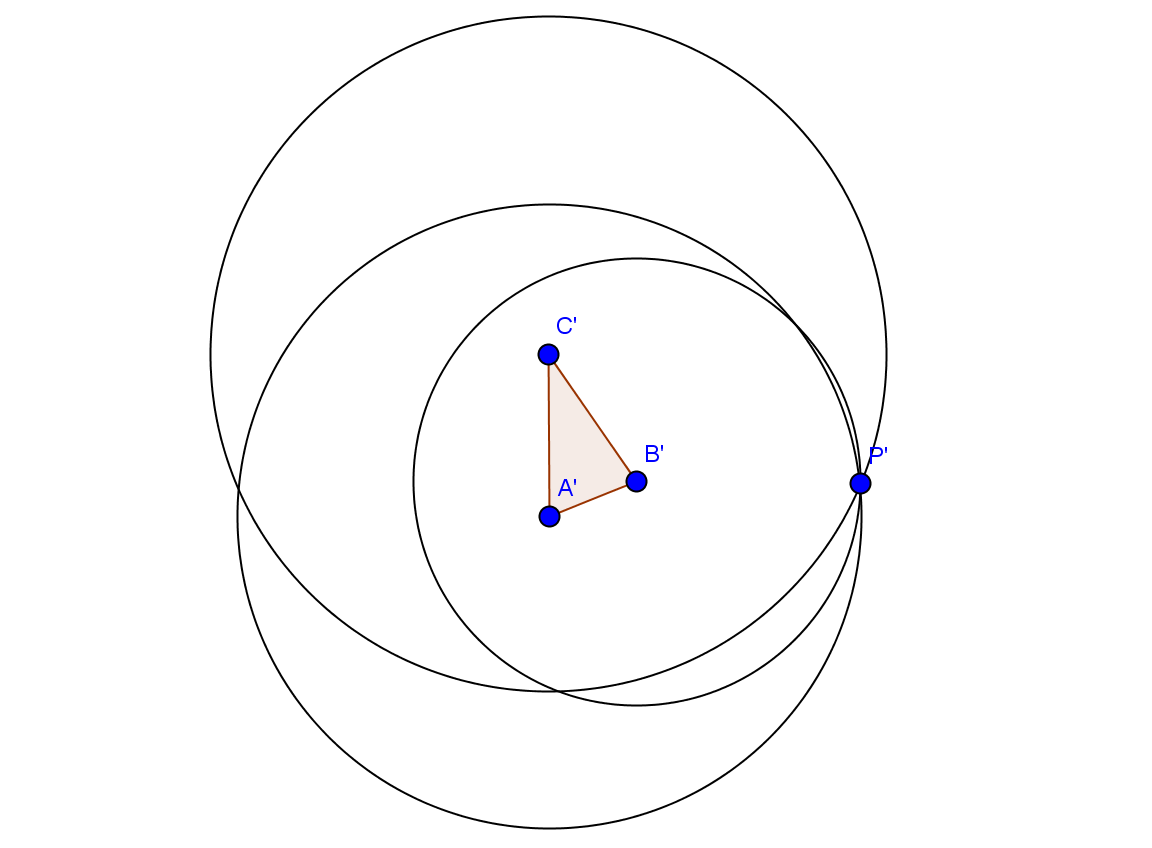Proposition: If T is an isometry, then T is 1:1 and onto as a function.

Proof: 1:1. Suppose that T(P)=T(Q). Then d( T(P),T(Q))=0=d(P,Q) so P=Q.

onto. Suppose R is in the plane. Consider A,B, and C in the plane where C is not on the line AB. Then the points T(A),T(B), and T(C) form a triangle and using the distances d(T(A),R), d(T(B),R), and d(T(C),R), we can determine a unique point X in the plane where d(A,X)=d(T(A),R), d(B,X) = d(T(B),R), and d(C,X)=d(T(C),R), so T(X) = R.

Now consider Euclid's treatment of the side-angle-side congruence [Proposition 4] and how it relates to transformations of the plane that preserve lengths and angles.

Such a transformation T: plane -> plane, has T(P)=P', T(Q)=Q' and T(R)=R' with d(P,Q) = d(P',Q') [distance between points are preserved] or m(PQ)=m(P'Q')  [measures of line segments are invariant].

Review briefly the outline of Euclid's argument  for Proposition 4.

Notes:

• The Side-side-side (SSS) congruence of triangles

• (If Corresponding sides of two triangles are congruent, then the triangles are congruent)
• This allows one to conclude that any isometry also transforms an angle to a congruent angle.
• The key connection between the congruence of figures in the plane and isometries:

• Proposition: Figures F and G are congruent if and only if there is an isometry of the plane T so that T(F) = {P' in the plane where P'= T(P) for some P in F} = G.
You can read more about isometries by checking out this web site: Introduction to Isometries.

We begin a more detailed study of isometries with a look at
Line Isometries:
Consider briefly isometries of  a  line.
1) translations and 2) reflections.
How can we visualize them?
• Mapping Diagrams (before and after lines) T: P -> P';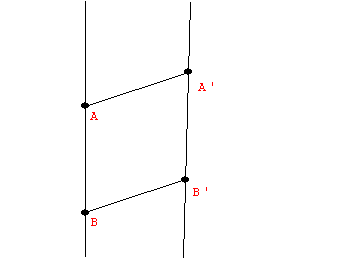Correspondence figures on a single line: Example: A reflection.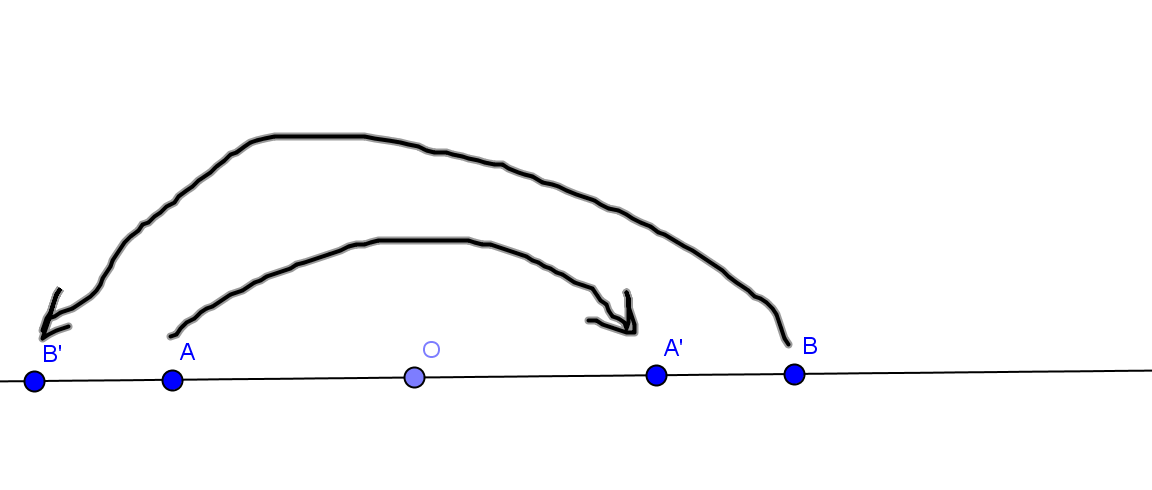Graph of transformation. Example: A reflection.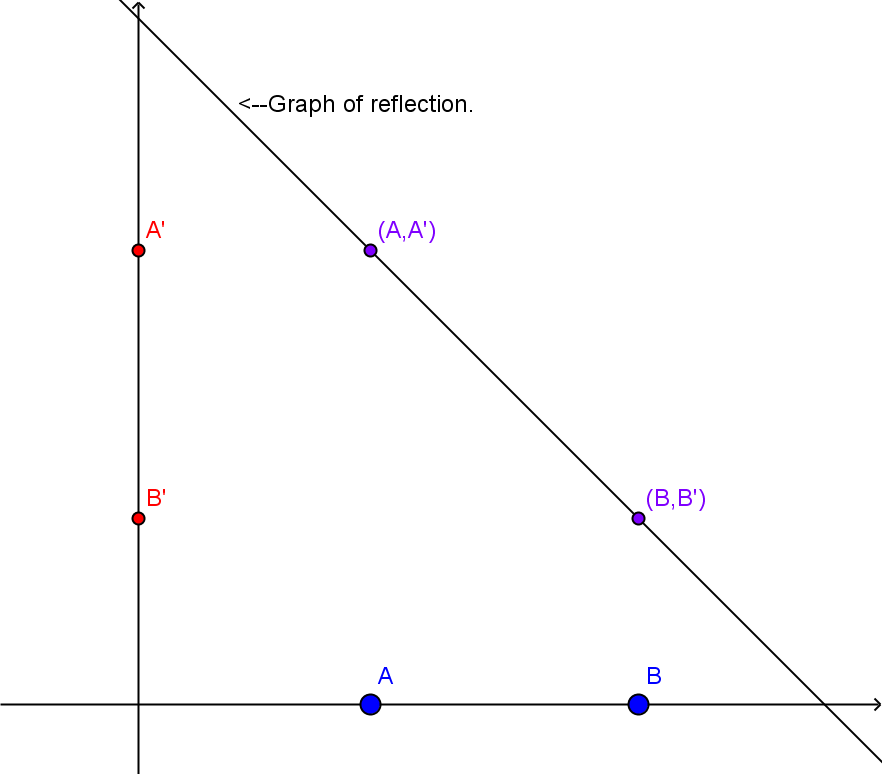2-29

Coordinate function. x -> x' = f (x)
Examples: P x -> Px+5 a translation; P x -> P-x  a reflection.

Can we classify them? Is every line isometry either a translation or a reflection? Why?

Prop.: The only isometries of the line are reflections and translations.
Proof:
Given A and A', there are only two choices for B '. One forces the isometry to be a translation, the other forces the isometry to be a reflection.

Discuss further in class.

Line isometries and coordinates:

Use T to denote both the geometric transformation  and the corresponding function transforming the coordinates of the points. So ... T(x) = x + 5 for the translation example and T(x) = -for the reflection example.
More generally, a translation
Ta :P x -> Px+a would have Ta(x) = x + a and Reflection about the origin can be denoted R0 , R0(x) = -x . What about a general reflection about the point with coordinate c? Rc(x) =?  Use a translation by -c, then reflect about 0, and translate back to c. So  Rc(x) = Tc(R0(T-c(x))) = Tc(R0((x -c))=Tc(-x+c)= -x + 2c.

Remarks on line isometries:
(i) Any isometry of a line can be expressed as the product of at most 2 reflections.
(ii) The product of two line reflections is a translation.

Now we look at Plane Isometries:
Consider isometries of  a  plane.
1) translations 2) rotations and 3) reflections.
How can we visualize them?

• Mapping Diagrams (before and after planes); [GeoGebra] [Add figures here.]
• Correspondence figures on a single plane; [GeoGebra]
• Graph? [Visualizing 4 dimensions! ]

Coordinate functions?
Remark: we have previously shown that an isometry of the plane is completely determined by the correspondence of three non-colinear points.

The classification of isometries.
There are (at least) f
our types of isometries of the plane: translation, rotation, reflection and glide reflection. [In fact , we will show that any planar isometry is one of these four types.]

• 3-2

• Proposition: Any plane isometry  is either a reflection or  the product of two or three reflections.
• Proposition:
(i) The product of two reflections that have the lines of reflection intersect at a point O is a rotation with center O through an angle twice the size of the angle between the two lines of reflection.

(ii) The product of two reflections that have parallel lines of reflection is a translation in the direction perpendicular to the two lines and by a length twice the distance between the two lines of reflection.

(iii) The product of three reflections is either a reflection or a glide reflection.

Proof
:
We can do this geometrically or using analytic geometry and the matrices!

• Note: The four types of isometries can be characterized completely by the properties of orientation preservation/reversal and the existence of fixed points. This is represented in the following table:
 Orientation  Preserving Orientation  Reversing Fixed points Rotations Reflections No Fixed points Translations Glide reflections

Note: The plane isometries form a group: A set together with an operation (the product or composition) that is
(0) Closed under the operation - the product of two isometries is an isometry;
(1) The operation is associative - R(ST) = (RS)T  or for any point P,  (R(ST))(P) = R( ST(P))= R(S(T(P))) = (RS)(T(P)) = ((RS)T)(P).
(2) The identity transformation (I) is an isometry -  I(P)=P.
(3) For any isometry T there is an (inverse) isometry S so TS = ST = I.
The operation of composition is not necessarily commutative in the sense that ST is not always the same transformation as TS:
For example If R1 and R2 are reflections in intersecting lines l1 and l2 then the isometry R1R2 is a rotation about the point of intersection in the opposite direction to R2R1.

• What about coordinates and plane isometries?

• Coordinates: (a la M&I I.3) Use any two non-parallel lines in the plane with coincident 0. Then you can determine "coordinates" for any point by using parallelograms. [As indicated previously, all rational coordinates can be constructed from establishing a unit. This is outlined more thoroughly in the reading in I.3.]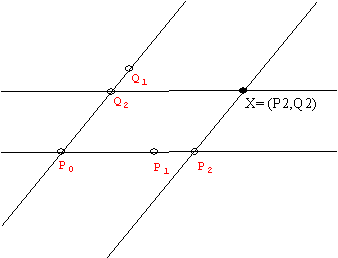Isometry examples with coordinates in the plane: (See M&I I.5 and I.6)

Translation: T: P (x,y) -> P(x+5, y+2) is a translation of the plane by the vector <5,2>. If we use the coordinates for the point and T(x,y) = (x',y') then x' = x+5 and y' = y+2.  We can express this with vectors <x',y'> = <x,y> + <5,2>. So translation corresponds algebraically to the addition of a constant vector.
Reflections: Across X-axis RX(x,y) = (x,-y); Across Y axis RY(x,y) = (-x,y);  Across Y=X , R(x,y)=(y,x). Notice that these can be accomplished using a matrix operation. Writing the vectors as row vectors

(x,y) (
 1 0 0 -1

) = (x,-y)
Matrix for RX
(x,y) (
 -1 0 0 1

) = (-x,y)
Matrix for RY
(x,y) (
 0 1 1 0

) = (y,x)
Matrix for R

Or writing the vectors as column vectors

 Matrix for RX [ 1 0 ] [ x ] = [ x ] 0 -1 y -y
and
 Matrix for RY [ -1 0 ] [ x ] = [ -x ] 0 1 y y
and
 Matrix for R [ 0 1 ] [ x ] = [ y ] 1 0 y x

Rotations:
If R90 is rotation about (0,0) by 90 degrees, then R90(x,y) = (-y,x).
Question: What is rotation about (0,0) by t degrees: R(t)?
Hint: What does the rotation do to the points (1,0) and (0,1)? Is this rotation a "linear transformation?"
More general Question: What about reflection R(A,B) about the line AX+BY = 0?

 Matrix for R90 [ 0 -1 ] [ x ] = [ -y ] 1 0 y x

 R(t):Matrix for rotation by t degrees? [ a b ] [ x ] = [ ax+by ] c d y cx+dy

Hint:
Consider that P(1,0) will be transformed to
P'(cos(t), sin(t))= (a,c
and Q(0,1) will be transformed to
Q'(-sin(t), cos(t))=(b,d)

 Matrix for R(A,B)? [ a b ] [ x ] = [ ax+by ] c d y cx+dy

3-4
More general Question: What about reflection R(A,B) about the line AX+BY = 0?

 R(t):Matrix for rotation by t degrees. [ cos(t) -sin(t) ] [ x ] = [ xcos(t)-ysin(t) ] sin(t) cos(t) y xsin(t)+ycos(t)

See discussion above.

 Matrix for R(A,B)? [ a b ] [ x ] = [ ax+by ] c d y cx+dy
Hint: Rotate, reflect, and rotate back!
Composition of Isometries corresponds to Matrix multiplication!

If B = 0 then the line of reflection is the Y axis and we know the matrix for RY already. For all other B, let t be the angle which has tan(t) = - A/B. Thus the reflection R(A,B) has a matrix that must be the product [from left to right]of the matrices for  R(t), RX, and R(-t).

 [ cos(t) -sin(t) ] [ 1 0 ] [ cos(t) sin(t) ]=[ cos2(t)-sin2(t) 2cos(t)sin(t) ]=[ cos(2t) sin(2t) ] sin(t) cos(t) 0 -1 -sin(t) cos(t) 2cos(t)sin(t) sin2(t)-cos2(t) sin(2t) -cos(2t)

Here is the visualization of R(A,B) as a map in Winplot using: (x,y)==>(cos(2t)x+sin(2t)y,sin(2t)x-cos(2t)y)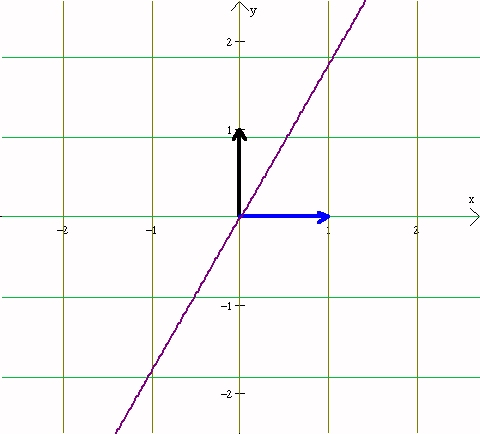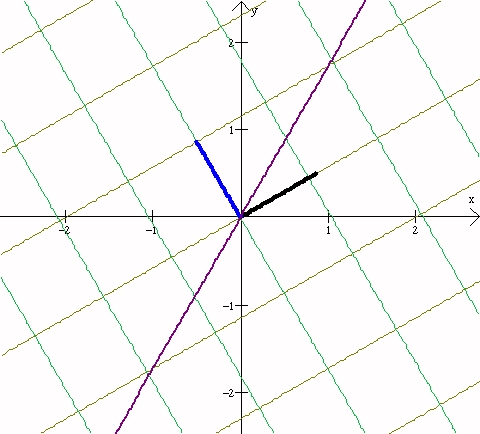Before Reflection After Reflection

More General Planar Isometries:
As with line isometries, a key idea is do the work at the origin and then transfer the work elsewhere using "conjugacy":  Any transformation T at a general point or about a general line can be investigated by first translating the problem to the origin, S, performing the related transformation at the origin,T',  and then translating the result back to the original position,
S-1. That is using the "conjugacy" operation: T = S-1 T' S  where T' is the relevant transformation at the origin.  This works as well for rotation and reflections through the origin because the composition of these transformations corresponds to matrix multiplication.

3-7
Here is a link to an example of the matrix for
the isometry of the coordinate plane that is rotation by 90 degrees counterclockwise about the point (1,2).

An Applications of Reflection:
(i) Here is a problem  encountered frequently in first semester of a calculus course.
The Carom Problem Can you see how the solution is related to a "carom" (angle of incidence=angle of reflection)?
(ii) Here is a similar problem about triangles that is also related to reflections.
Fano's Problem  Can you find the relationship?

Symmetry of a figure: S is a symmetry of a plane figure F  if S is an isometry with S(F)=F. Given a figure F, the symmetries of F form a subgroup of all the plane isometries, denoted Sym(F).
Example: Consider the figure F= an given equilateral triangle. We looked at the six symmetries of this figure and the table indicating the multiplication for these six isometries.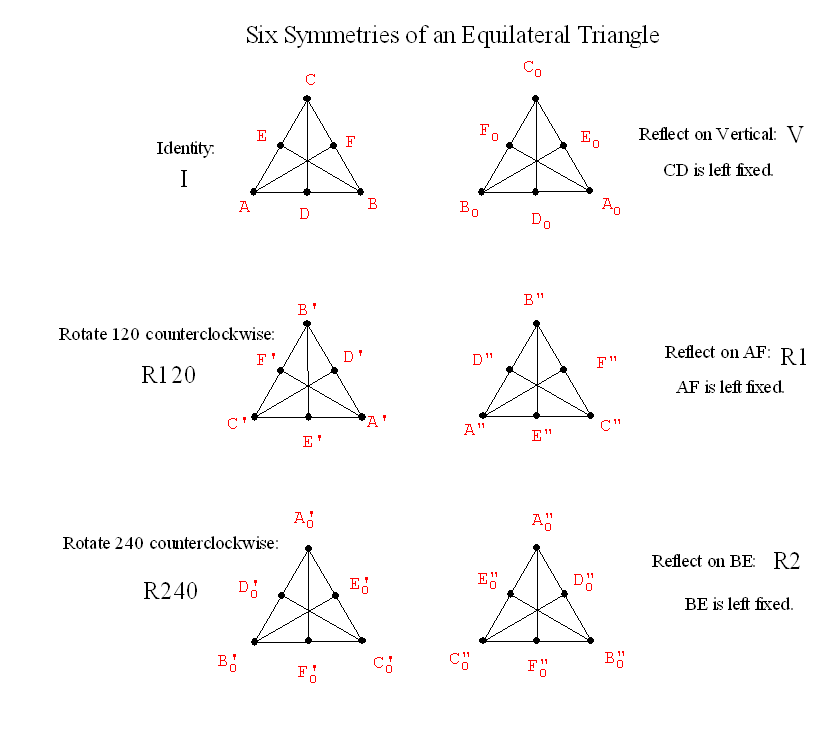R*C I R120 R240 V R1 R2 I I R120 R240 V R1 R2 R120 R120 R240 I R2 V R1 R240 R240 I R120 R1 R2 V V V R1 R2 I R120 R240 R1 R1 R2 V R240 I R120 R2 R2 V R1 R120 R240 I
Two interesting sites for looking at symmetries are the Symmetry Web page  and the Symmetry, Crystals and Polyhedra page.
• Discussion: What about isometries in three dimensions?

• These are generated by spatial reflections in a plane.
A spatial isometry is determined by the transformation of 4 points not all in the same plane (which determine the simplest 3 dimensional figure- a tetrahdron!)
Any isometry of space can be expressed as the product of at most 4 reflections.
These results are proven in the same fashion as the comparable results were proven in the plane.

• Proportions and similarity:
An example of the use of similar triangles and proportions to constructing "square roots":
Mean Proportions in right triangles and Inverses:
Consider a right triangle ABC with hypotenuse AB. Notice that if the altitude CD is constructed with the hypotenuse AB as the base, the figure that results has 3 similar right triangles. ABC, ACD, and DCB. Using similarity of these triangles we see that there is a proportion of the segments of the hypotenuse AD, DB and the altitude CD given by AD:CD::CD:BD. If we consider the lengths of these segments respectively as a,h, and b then the numerical proportion may be expressed as a/h=h/b or using common algebra $ab=h^2$
. Notice this says that $h= \sqrt{ab}$.

Application of this construction: Choosing a = 1, this proportion becomes $h = \sqrtb$

A look at problems caused by the diagonal of a square and the issue of finding a unit that would measure both the side and the diagonal.
First look at the Euclidean algorithm for finding a common segment with which to measure two segments.
Euclid's original treatment of the "division algorithm" :
If  n and d>0 are integers
then there are integers q and r with r=0 or 0<r<d where n = q*d +r.

Using q*OD to represent a segment that is made of q segments all congruent to OD,
Euclid's division algorithm is stated in geometry that of ON is a segment and OD is a segment that is contained as a subsegment of ON, then ON is congruent to q*OD  with  possibly a remaining segment RN which is congruent to a subsegment of OD.

Repeated use of this algorithm suggests the Euclidean algorithm for finding a common unit to measure both n and d, or ON and OD.

If r1=0 or R1N is a point, then OD will be a common unit.
If not apply the division algorithm to d or OD and r1 or R1N.
If this works to give r2= 0 or R2N is a point, then R1N will be the common unit.
If not apply the division algorithm to r1 or R1N and r2 or R2N.
If this works to give r3= 0 or R3N is a point, then R2N will be the common unit.
If not continue.
In common arithmetic since each remainder that is not zero is smaller than the previous remainder, eventually the remainder must be 0 and the process will end- finding a common divisor of the original d and n.

In the application of the euclidean algorithm to the diagonal and side of a square, the procedure appears to stop.
However, we can show that because of the fundamental theorem of arithmetic, it would be impossible to find a segment with which to measure both the side and the diagonal of a square.

The impact of this on geometry was that one could not presume that all of geometry could be handled by using simple ratios of whole numbers for measurements.

[A geometry based on ratios alone would not permit one to accomplish proposition 1 of Book I of Euclid! since this would mean that the geometry would have to be able to have ratio involving the sqr(3) - which like the sqr(2) is also an irrational number.]

3-9
A major part of geometry before Descartes was Euclid's (Eudoxus') resolution of the issue in Book V def'ns 1-5.(Joyce)
Definition 1
A magnitude is a part of a magnitude, the less of the greater, when it measures the greater.
Definition 2
The greater is a multiple of the less when it is measured by the less.
Definition 3
A ratio is a sort of relation in respect of size between two magnitudes of the same kind.
Definition 4
Magnitudes are said to have a ratio to one another which can, when multiplied, exceed one another.
Definition 5 (Byne's)
Magnitudes are said to be in the same ratio, the first to the second and the third to the fourth, when,
if any equimultiples whatever are taken of the first and third,
and any equimultiples whatever of the second and fourth,
the former equimultiples alike exceed, are alike equal to, or alike fall short of, the latter equimultiples respectively taken in corresponding order.

Look at these definitions and note some key items:
* Ratios exist only between magnitudes of the same type. (This is usually described as "Homogeneity".)
* For ratios to be equal the magnitudes must be capable of co-measuring.
* Euclid's axioms do not deny the existence of infinitesimals- but will not discuss equality of ratios that use them.

Briefly: An infinitesimal segment is a segment AB that is so short that it would appear to be coincident points and for any k, k*AB cannot contain any ordinary segment determined by points that we can see as distinct!
I
n the history of mathematics, infinitesimal segments we used in the early developments of the calculus. For example: Consider the ratio of {the change in the area of a square, A(s), when the length of a side, s, is changed by an infinitesimal, ds} to {the change in the length of the side, ds}. That is: What is [A(s + ds) - A(s)]/ds ?  Answer: [2s*ds + ds*ds]/ds = 2s +ds. Thus the ratio would be indistinguishable as a number from 2s. ]

* The  Axiom of Archimedes that says that for any two segments one can be used  to measure the other. [There are no infinitesimals for an Archimedean geometry.]

3-21 Example: Euclid uses the theory of proportion in Book VI, Proposition 1 and Proposition 2.

The connection [between Euclid's definition of proportionality (equal ratios) and real number equality of quotients].
We can show the following
Proposition: For segments A,B,C,and D with m(A)=a,m(B)=b, m(C)=c, and m(D)=d:
1. If A:B::C:D then a/b=c/d .
2. If a/b=c/d then A:B::C:D
Proof.

• The concept of similarity as a transformation.

• Euclidean Constuction for a Central Similarity:

Proposition 1 (Book VI) of Euclid provides the tool for the central similarity transformation in Euclidean geometry.  To perform the transformation of the plane with center O and using two given line segments to determine the magnification, first draw a ray from O and locate P and P' on the ray so the given segments are congruent to OP and OP'. To transform a point Q
draw the ray OQ, then the segment PQ. Through P' construct a line l that is parallel
to PQ. Then l will meet OQ at a point Q' and by proposition 1, Q' is the appropriate point to which Q is transformed by the central similarity as required.

It is not difficult to show from this construction that a central similarity will transform a line  l to a line l' that is parallel to the original line l. Thus one can prove the related results for euclidean geometry:
Corollary: If T is a central similarity then
(i) T preserves the measure of angles and
(ii) T transforms a pair of parallel lines to parallel lines.

Since isometries also preserve the measure of angles and transform a pair of parallel lines to parallel lines (since a reflection does) we see that any composition of central similarities and isometries will likewise preserve the measure of angles and transform a pair of parallel lines to a parallel lines.
Exercise: Show such compositions will transform a triangle to a similar triangle and ABC and A'B'C' is any pair of similar triangles, the there is an isometry T and a central similarity S so that TS transforms ABC to A'B'C'.

Interlude (not covered in Lecture): Using Vectors in a geometric structure:
The segment connecting midpoints of the sides of a triangle proposition: A Vector Proof

Similarity Transformations in Coordinate Geometry. [Not covered in class.]
Consider a similarity on a line with a center of similarity and a given positive magnification factor. This leads to a consideration of the effect of a similarity on the coordinates of a point on the line.
If we use the point P0 for the center, then we see that the similarity T with magnification factor of 2 would transform Px to P2x, or T(Px)=P2x. Removing the P from the notation we have T(x)=2x. Using T(Px)=Px', we find that T is described by the correspondence where x'=2x.

[More generally: If we use the point P0 for the center, then we see that the similarity T with magnification factor of m would transform Px to Pmx, or T(Px)=Pmx. Removing the P from the notation we have T(x)=mx. Using T(Px)=Px', we find that T is described by the correspondence where x'=mx. ]

With the center at another point, say P3, the transformation T* is controlled by the fact that T*(Px) - P3 = 2(Px-P3).
So that  for T* we have x' = 3 + 2(x-3).

[Again generally: With the center at point Pa, the transformation T* is controlled by the fact that T*(Px) - Pa = m(Px-Pa). So that  for T* we have x' = a + m(x-a).]

We can also observe that if we let S(Px)=P(x-3) and S-1(Px)=P(x+3) then

S-1(T(S(Px))) = S-1(T(P(x-3)))
= S-1(P2(x-3))
= P(3+2(x-3))
= T*(Px),
so S-1TS=T*.

Central similarities in the plane:
In the plane, a similarity Tm  with factor m and center at (0,0) will transform  (x,y) to (mx,my). Thus x' = mx, and y' = my are the equations for this transformation. This transformation can be represented using a matrix as follows:

 Matrix for Tm [ m 0 ] [ x ] = [ mx ] 0 m y my
Other central similarities T* with factor m and center at (a,b) can be recognized as related to Tm by using the translation S(x,y) = (x - a,y - b) and seeing that  S-1TmS=T*.
[Try this yourself!]

Proposition: If l is a line in the plane with equation AX+BY=C  with A and B not both 0, then the set l' = {(x',y'): Tm(x,y)=(x',y') for some (x,y) on l} is a line in the plane that is parallel to l (unless l = l' ).
Proof (outline): Show that the equation of l' is  AX+BY = mC. Suppose (x,y) satisfies the equation
AX+BY=C. Then Tm(x,y) = (mx,my).  But Amx+Bmy=m(Ax+By)=mC, so the line l ' has equation AX+BY=mC, and therefore is parallel to the line l.

We can use coordinate geometry to describe a central similarity S of factor 5 with center at (3,2)  as  follows: (x,y) -> (x - 3,y - 2) -> (5(x -3) ,5(y - 2))  -> (5(x -3) +3 ,5(y - 2) +2).
Thus S
(x , y) = (5x -12 ,5y - 8).
Here is the visualization of S(x,y) as a map in Winplot:Before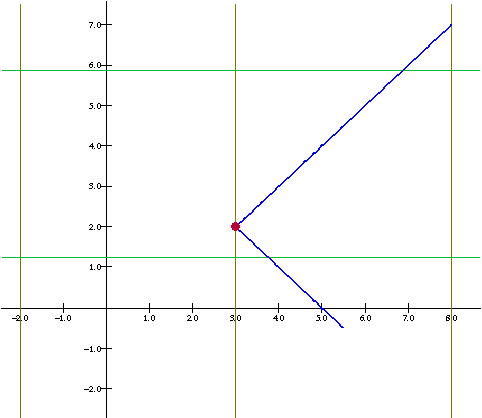After

View video (in Library #4376 ) on "Central similarities" from the Geometry Film Series. (10 minutes)
View video (in Library #209 cass.2) on similarity (How big is too big? "scale and form")  "On Size and Shape"  from the For All Practical Purposes Series. (about 30 minutes)

The Affine Plane: A first look at an alternative geometry structure for parallel lines.